Signal Processing > Fourier Transform PropertiesShowing results 1 to 10 of 11, on page 1 of 2
 DESCRIPTION EQUATION Discrete-Time Fourier frequency differentiation propertyDiscrete-Time Fourier frequency shift property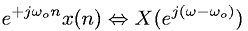Discrete-Time Fourier linearity theorem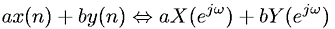Discrete-Time Fourier time reversal property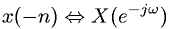Discrete-Time Fourier time reversal property; x(n) realDiscrete-Time Fourier time shift propertyDiscrete-Time Fourier time/space convolution property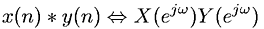Discrete-Time Fourier transform - Parseval's Theorem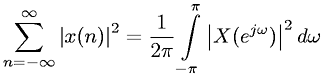Discrete-Time Fourier transform - Parseval's TheoremDiscrete-Time Fourier transform definitionGo to page: 1  2